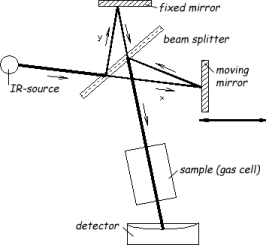• ### What is an “FTIR” analyser?

Date posted:

• Post Author

### 1. General

A FTIR (Fourier Transform Infrared) – analyser is an instrument for (IR) [GLOSS]infrared[/GLOSS] spectroscopy. It allows the qualitative and the quantitative measurement of a lot of polar substances, e.g. the gases CO2, CO, CH4, NH3, HCN, NO, N2O, NO2, SO2, HCl, etc. Thereby the FTIR – analyser uses – in opposite of the conventional, dispersive IR spectrometer – an interferometer and [GLOSS]Fourier transformation[/GLOSS]. Because of this, there are following advantages to the conventional, dispersive IR spectrometer:

• strong reduction of the measuring time, because of the simultaneous detection of IR radiation of all wave numbers during the hole measuring time (mulitplexer advantage). The FTIR technique allows scan speeds up to 1 spectra per second whereas IR analyzer with monochromators need 1 minute or longer to take one spectra.
• FTIR-analyzers are not using monochromators (devices which just allow the throughput of light with a special wavelength) almost the complete light emitted (just reduced by losses by mirrors, sample material, etc. ) arrives at the detector. A high IR-light intensity on the detector is leading to a high SNR (Signal-Noise-Ratio) because a linear increase of the light intensity effects a quadratical increase of the SNR. This high light throughput is a result of the interferometer technique and is called Jacquinot advantage.
Since the interferometer was introduced (in the 1970s) to the IR spectroscopy, its importance increased as universal analysis for organic and inorganic samples.

### 2. The theory of infrared spectroscopy

In the IR spectroscopy the interaction between matter and electromagnetic radiation is used. IR light can be absorbed by molecules, whereby the dipole moment of the molecule is changed which effects molecular vibrations. Requirements for this are that the molecule has a dipole moment and that the frequency of molecular vibration and IR radiation are equal. This frequency again depends on the mass of the vibrating atom and its chemical bond strength.

The simplest model of a vibrating molecule describes an atom bond to a very large mass by a weightless spring. The force F which is necessary to move the atom by a certain distance x from an equilibrium position is proportional to the force constant k, a measure of the strength of the bond, and is equal to the force of the spring to return to the equilibrium position. This is Hooke´s law (1):

F = -k x       (1)

The basic equation for IR spectroscopy (2) shows the dependence between frequency and the involved atoms and bonds. This also shows that different atom-bond-combinations vibrate in different frequencies and absorb IR radiation at different wavenumbers.

E = hn = (1/ 2p)( k/ R)0.5      (2)

where:
– F is the force (N)
– x is distance (m)
– k is the force constant (N/m)
– E is the energy
– h is the Planck’s constant (J s)
n is the frequency (1/s)
– R is the reduced mass (1/kg)

As a consequence, a molecule will absorb IR light only if the absorption causes a change in the dipole moment. Thus, all compounds except for elemental diatomic gases, such as N2, H2 have IR spectra and most components present in a flue gas can be analysed by their characteristic IR absorption.

### 3. The fourier transform infrared spectrometer

The main component of an FTIR spectrometer is the Michelson interferometer, as illustrated in Figure 1. The interferometer consists of a fixed mirror, a movable mirror, and a beamsplitter. The beamsplitter transmits half of the incident radiation to the moving mirror and reflects the other half to the fixed mirror. The two beams are reflected by these mirrors back to the beamsplitter, where they recombine. The optical path difference between the two beams is called the retardation d. When the fixed mirror and moving mirror are equidistant from the beamsplitter (i.e. y=x), the amplitude is in phase and recombines constructively, the retardation is zero. As a result, all the light from the source will reach the sample or detector. Displacement of the movable mirror will result in a retardation, and the beams will be out of phase causing a destructive interference. The function of the intensity versus the retardation is called interferogram.Figure 1: Schematic of a FTIR spectrometer

source: e.g. ceramic (Nernst stick),
beamsplitter: semi pervious mirror, e.g. KBr,
mirrors : gold or silver coated glass parts,
gas cell: preferably small volume and high optical pathlength by multiple reflections,
detector: e.g. DTGS-detector (deuterated triglycin sulfat) for standard applications and semiconductor detector, e.g. MCT-detector (mercury cadmium telluride) for higher sensitivity and scan speed for advanced demands.

The discrete interferogram is Fourier transformed by a PC to yield a wave number dependent spectrum.

For more details about the theoretical background of FTIR analysis see Schrader – Infrared and Raman Spectroscopy, VCH (1995).

### You may also like

• #### What is the relationship between fuel nitrogen and NOx emissions?

20th January 2003

• #### How is a sampling train prepared for sampling trace metals and particulates in flue gases?

16th December 2002